# Monopoly

Multiple Choice - Select best answer

Which of the following is false?
a. A monopolist will sell less at a higher price.
b. A monopolist will produce where MR = MC.
c. A monopolist has a marginal revenue that is less than the price.
d. A monopolist is a price taker.

A monopoly will usually produce
a. where its demand curve is elastic.
b. where its demand curve is inelastic.
c. only when its demand curve is perfectly inelastic.
d. where its demand curve is either elastic or inelastic.

Monopoly is characterized by
a. non-price competition not necessary.
b. market entry and exit difficult or impossible.
c. unique products.
d. All of the above

This Homework Help Question: "Monopoly" No answers yet.

We need 10 more requests to produce the answer to this homework help question. Share with your friends to get the answer faster!

0 /10 have requested the answer to this homework help question.

Once 10 people have made a request, the answer to this question will be available in 1-2 days.
All students who have requested the answer will be notified once they are available.

#### Earn Coin

Coins can be redeemed for fabulous gifts.

Similar Homework Help Questions
• ### ID: A 9. When a monopolist is able to sell its product at different prices, it...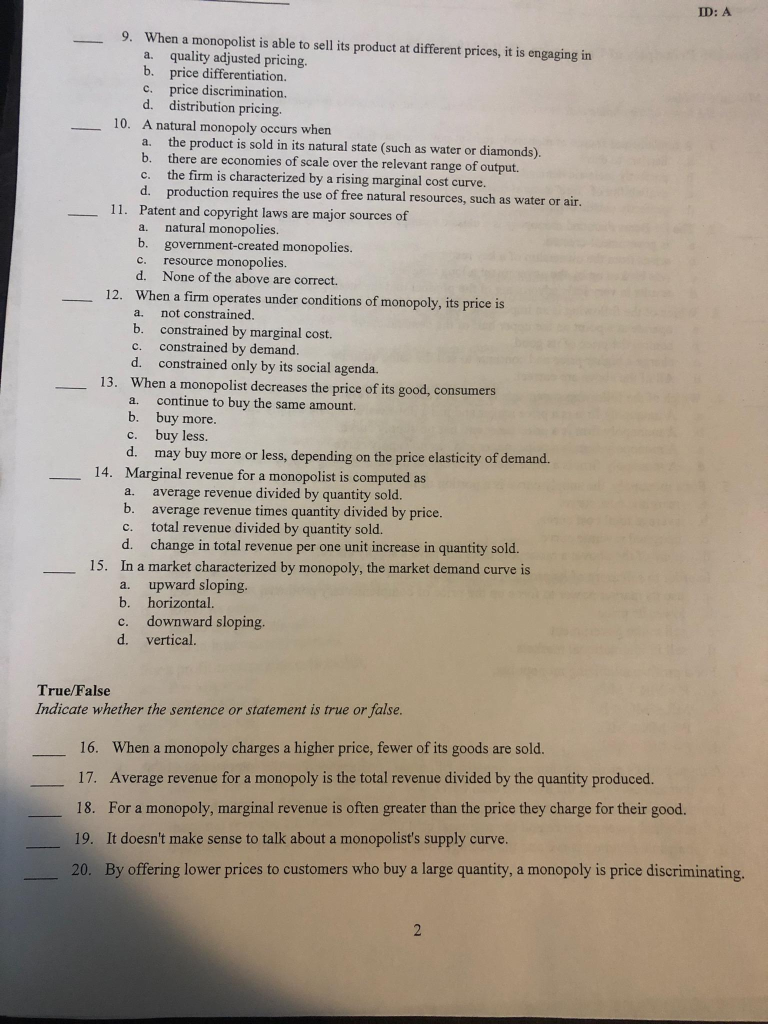ID: A 9. When a monopolist is able to sell its product at different prices, it is engaging in a quality adjusted pricing. b. price differentiation. c. price discrimination. d. distribution pricing. 10. A natural monopoly occurs when a. the product is sold in its natural state (such as water or diamonds). b. there are economies of scale over the relevant range of output. c. the firm is characterized by a rising marginal cost curve. d. production requires the use...

• ### 1. Assume that at a given level of output a monopoly firm has marginal revenue of...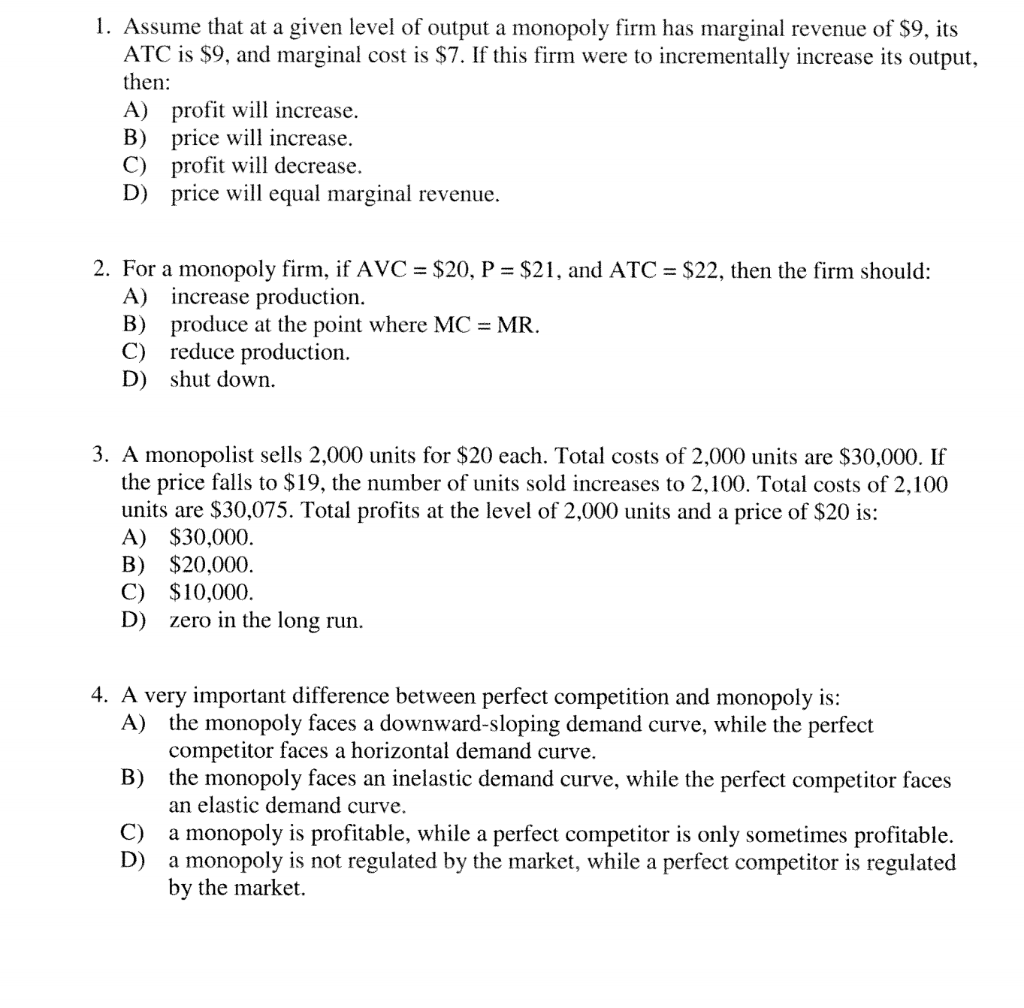1. Assume that at a given level of output a monopoly firm has marginal revenue of \$9, its ATC is \$9, and marginal cost is \$7. If this firm were to incrementally increase its output then A) profit will increase B) price will increase C) profit w decrease D) price will equal marginal revenue. 2. For a monopoly firm, if AVC = \$20, P = \$21, and ATC = \$22, then the firm should: A) increase production. B) produce at...

• ### A monopoly has A. A perfectly elastic demand curve B. A perfectly elastic supply curve C....

A monopoly has A. A perfectly elastic demand curve B. A perfectly elastic supply curve C. An inelastic demand curve D. less elastic demand curve than a competitive firm

• ### Consider the relationship between monopoly pricing and the price elasticity of demand.   If demand is...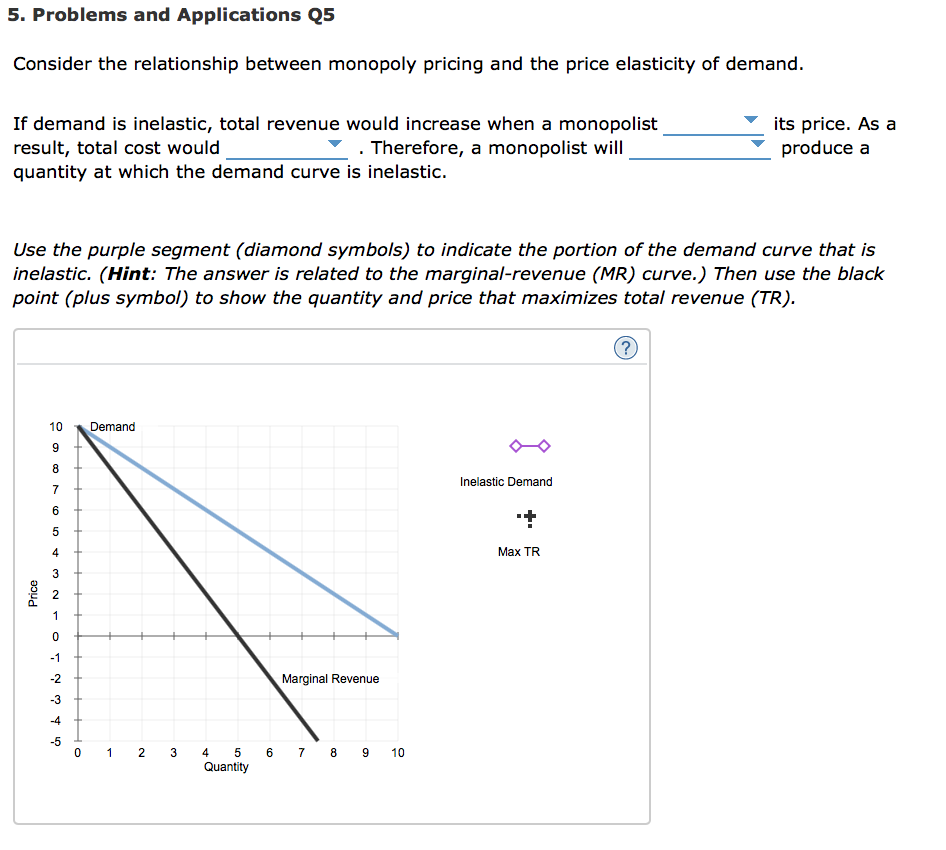Consider the relationship between monopoly pricing and the price elasticity of demand.   If demand is inelastic, total revenue would increase when a monopolist result, total cost would quantity at which the demand curve is inelastic. its price. As a produce a . Therefore, a monopolist will produce a quantity at which the demand curve is inelastic.   Use the purple segment (diamond symbols) to indicate the portion of the demand curve that is inelastic. (Hint: The answer is related...

• ### 1) Which of the following statements regarding a monopolist is correct? A) A monopolist will only...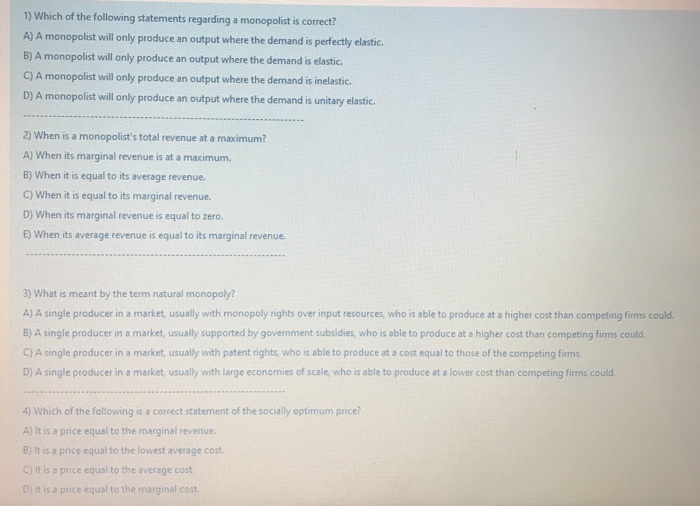1) Which of the following statements regarding a monopolist is correct? A) A monopolist will only produce an output where the demand is perfectly elastic, B) A monopolist will only produce an output where the demand is elastic C) A monopolist will only produce an output where the demand is inelastic. D) A monopolist will only produce an output where the demand is unitary elastic. 2) When is a monopolist's total revenue at a maximum? A) When its marginal revenue...

• ### NAME PRINT LAST NAME, FIRST NAME SECTIONE MONOPOLY is a pure monopoly when: İt is the...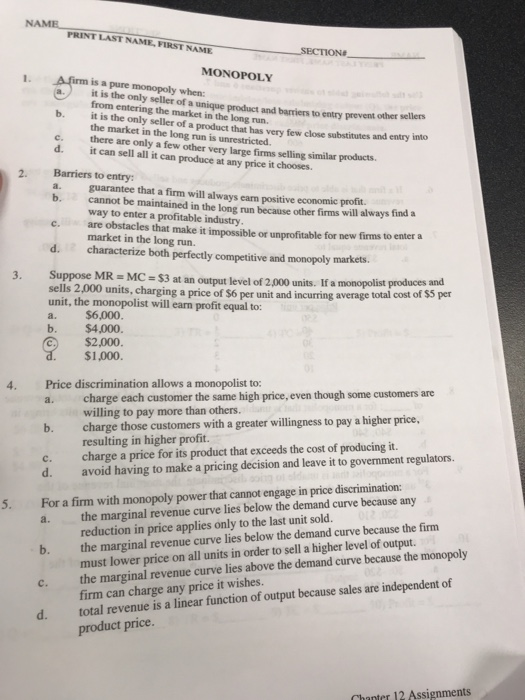NAME PRINT LAST NAME, FIRST NAME SECTIONE MONOPOLY is a pure monopoly when: İt is the only seller of a unique product and barriers to entry pneventother selen from entering the market in the long run it is the only seller of a product that has the market in the long run is unrestricted b. very few close substitutes and entry into C. there are only a few other very large firms selling similar products. d. it can sell all...

• ### PART III COVERS CLO 5 uan 4 marks Question 1 Choose the correct answer. Each question...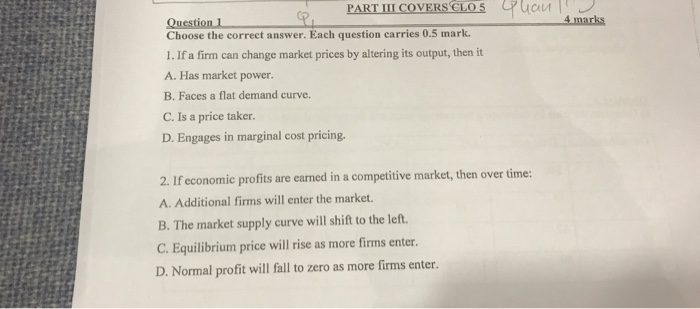PART III COVERS CLO 5 uan 4 marks Question 1 Choose the correct answer. Each question carries 0.5 mark 1. If a firm can change market prices by altering its output, then it A. Has market power. B. Faces a flat demand curve. C. Is a price taker D. Engages in marginal cost pricing. 2. If economic profits are earned in a competitive market, then over time: A. Additional firms will enter the market. B. The market supply curve will...

• ### 7. How is monopoly different from perfect competition? 8. What is a barrier to entry? Give...

7. How is monopoly different from perfect competition? 8. What is a barrier to entry? Give some examples. 9. What is a natural monopoly? 11. What is predatory pricing? 14. In what sense is a natural monopoly “natural”? 15. How is the demand curve perceived by a perfectly competitive firm different from the demand curve perceived by a monopolist? 16. How does the demand curve perceived by a monopolist compare with the market demand curve? 17. Is a monopolist a...

• ### Which of these statements regarding the differences between monopoly and a competitive market are true? Choose...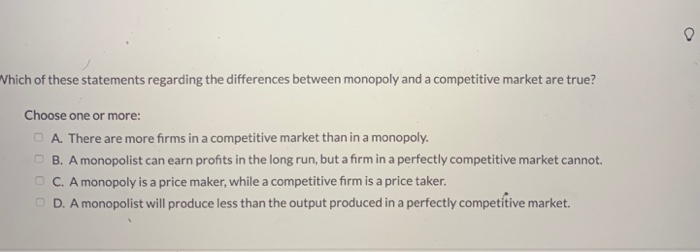Which of these statements regarding the differences between monopoly and a competitive market are true? Choose one or more: A. There are more firms in a competitive market than in a monopoly. B. A monopolist can earn profits in the long run, but a firm in a perfectly competitive market cannot. C. A monopoly is a price maker, while a competitive firm is a price taker. D. A monopolist will produce less than the output produced in a perfectly competitive market.

• ### please explain the answers 89. If a monopolist were to produce in the inelastic segment of...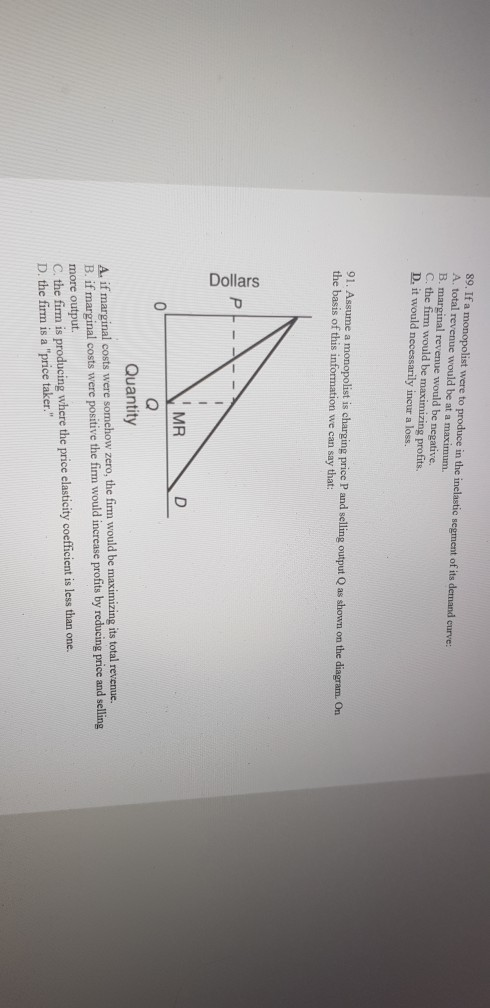please explain the answers 89. If a monopolist were to produce in the inelastic segment of its demand curve A. total revenue would be at a maximum. B. marginal revenue would be negative. C. the firm would be maximizing profits. D. it would necessarily incur a loss. 91. Assume a monopolist is charging price and selling output Q as shown on the diagram. On the basis of this information we can say that: Dollars MR 0 Quantity A. if marginal...